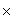From: Charlie, a student What is the correct formula for finding the answer for the following question? Selling a piece of furniture for \$670 and the profit is 35%. What is the original cost? Hi Charlie. The selling price equals the original cost plus the profit. Let's show this with variables: S = C + P You also know that the profit it 35% of the cost. That means: P = 35%C Now you can substitute this expression for P into the first equation: S = C + (35%C) You know S = \$670, so now you just need to solve the equation for C. I'm pretty sure you can handle it from here, Charlie. Stephen La Rocque. >